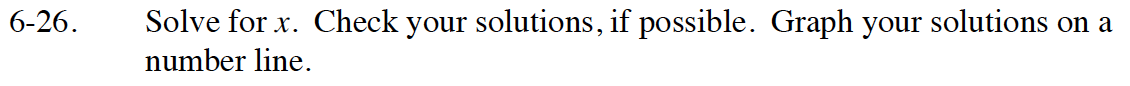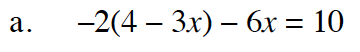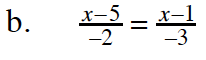Home > GB8I > Chapter cc46 > Lesson cc46.1.3 > Problem6-26

6-26.
1. Solve for x. Check your solutions, if possible. Graph your solutions on a number line. Homework Help ✎

1. −2(4 − 3x) − 6x = 10

2.Distribute the −2.

−8 + 6x − 6x = 10

Simplify.

−8 = 10

Start by multiplying both sides by a common multiple to remove the fractions.

There is no solution to this equation, because −8 ≠ 10.